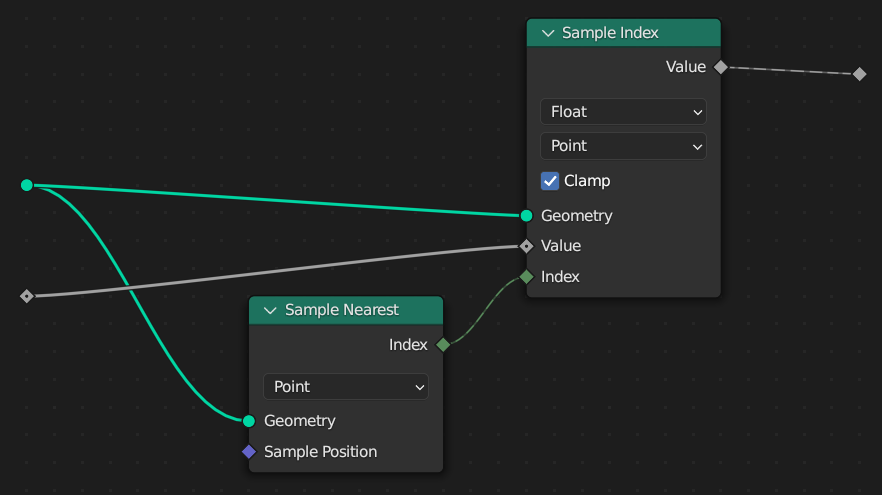# Sample Nearest Node

The Sample Nearest node retrieves the index of the geometry element in its input geometry that is closest to the input position.

This node is similar to the Geometry Proximity Node, but it outputs the index of the closest element instead of its distance from the current location.

Tip

If you want to find nearest to each point in same geometry, its better to use the Index of Nearest node.

## Inputs

Geometry

The geometry to sample.

Note

This node only supports point cloud and mesh inputs.

Sample Position

The position to start from when finding the closest location on the target geometry. By default, this is the same as if the Position Node was connected.

## Properties

Domain

The attribute domain to consider the distance from.

## Outputs

Index

The index of the closest geometry element of the chosen domain.

## ExamplesCombining this node with the Sample Index Node gives a setup that can retrieve the closest attribute value from another geometry. This is the same behavior as the Transfer Attribute node in versions of Blender before 3.4.